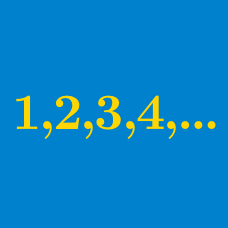Algebra

# Arithmetic Mean

What is the arithmetic mean of $3a +15$ and $7a -9$?

$8, x, 16, 18$

If the average of the above numbers is 13, what is $x$?

What is the arithmetic mean of all the prime numbers less than 20?

What is the arithmetic mean of all the leap years in the $19^{\text{th}}$ century?

Details and Assumptions:
A year that is divisible by 4 is a leap year. However, a year that is divisible by 100 but not by 400 is a common year.

The $19^{\text{th}}$ begins with January 1, 1801 and ends with December 31, 1900.

$-10, -6, -2, 2, 6, 10$ What is the arithmetic mean of the above sequence?

×